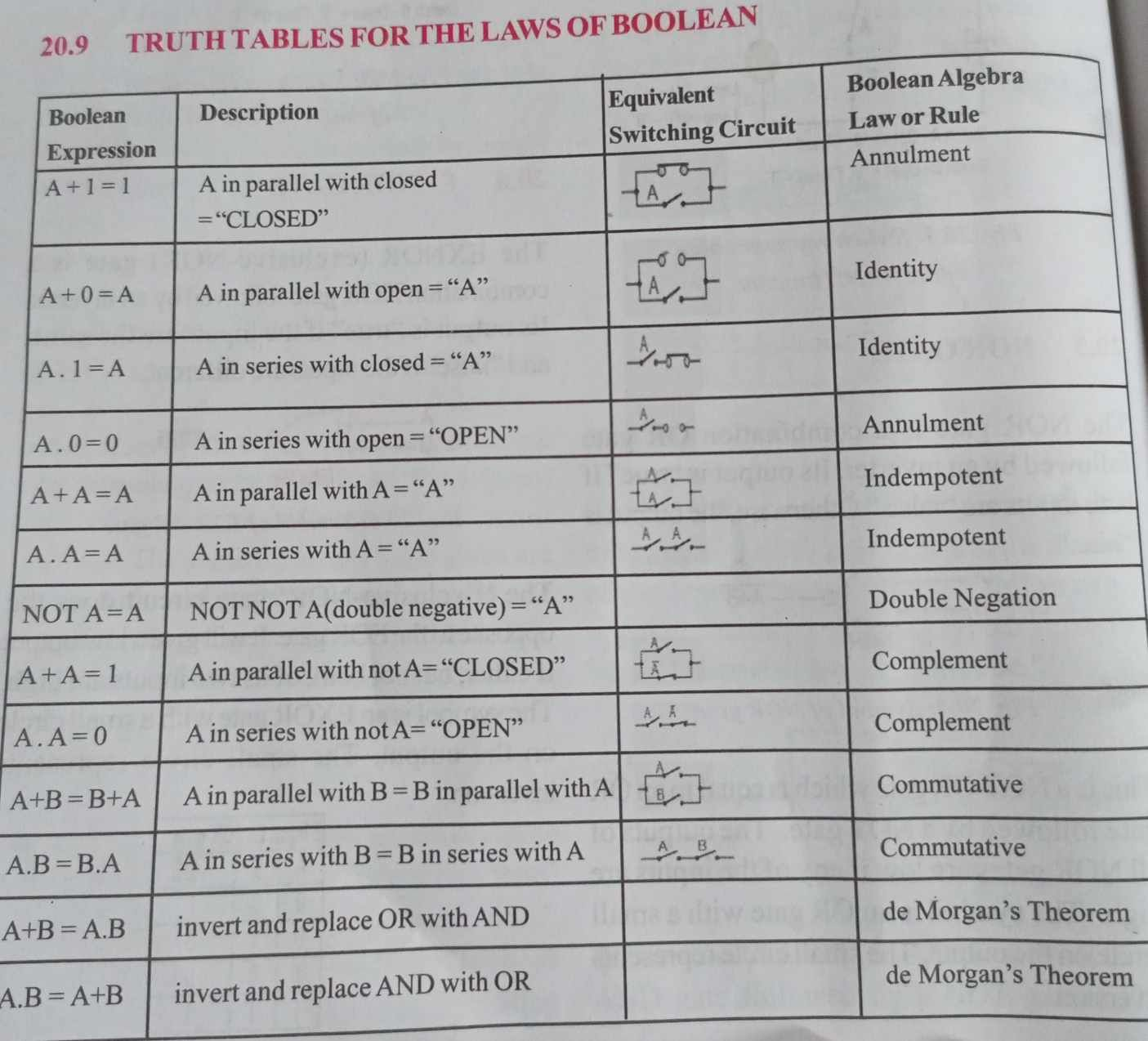Home » ALTERNATIVE LOGIC GATE

# ALTERNATIVE LOGIC GATEA logic gate is a general purpose electronic device used to construct logic circuits. All logic gates have inputs and outputs. The state of the output is set by the input states using different rules depending on the type of gate. The different types of gates have different shaped circuit symbols. The standard single logic gates are AND, OR and NOT (Inverter) and the alternative logic gates are exclusive – OR, exclusive – NOR, NAND and NOR gates.

EXOR GATE

The XOR (exclusive-OR) gate acts in the same way as the logical “either/or.” The output is “true” if either, but not both, of the inputs are “true.” The output is “false” if both inputs are “false” or if both inputs are “true.” Another way of looking at this circuit is to observe that the output is 1 if the inputs are different, but 0 if the inputs are the same.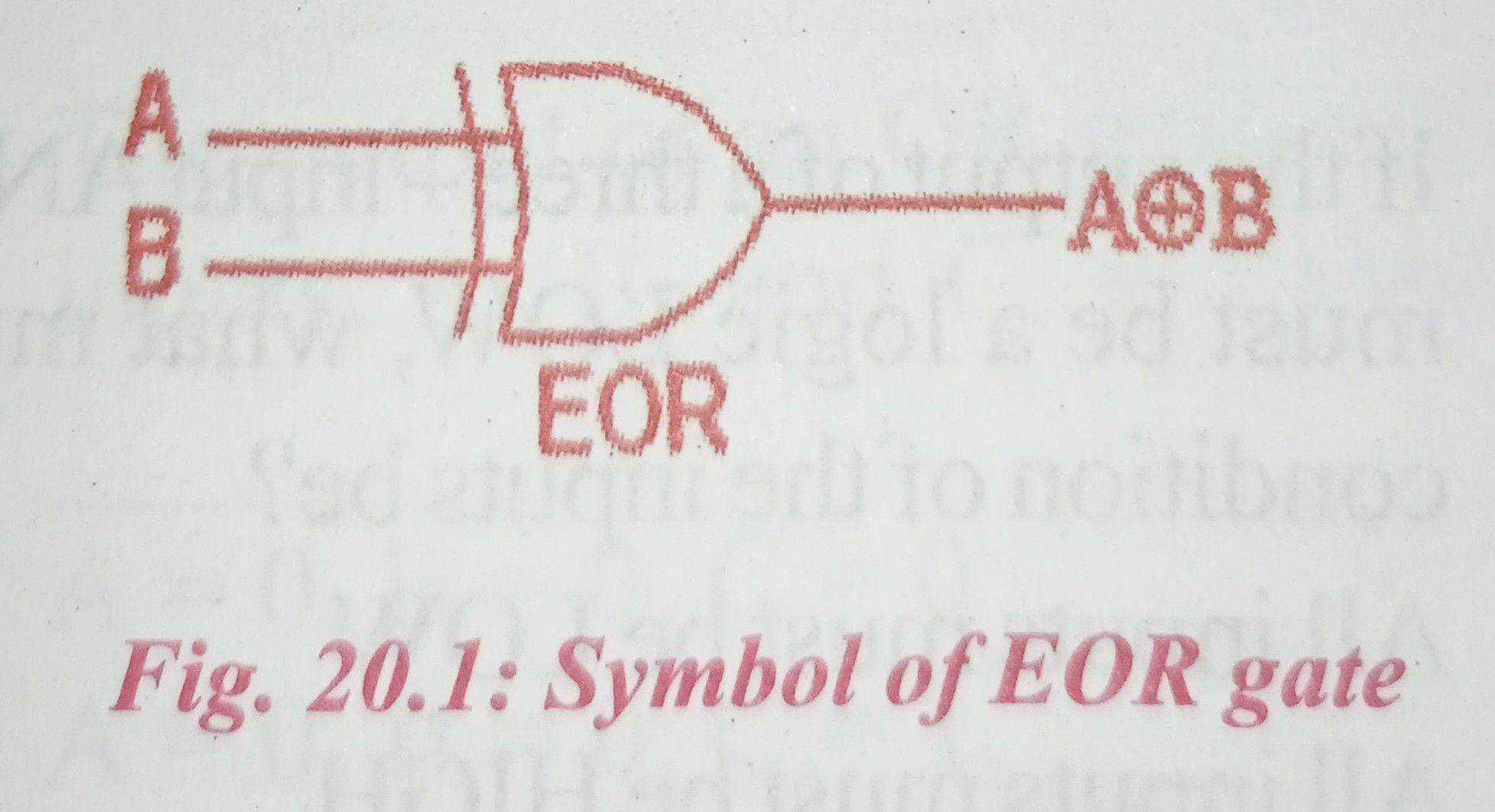The ‘Exclusive-OR’ gate is a circuit which will give a high output if either, but not both, of its two inputs are high. An encircled plus sign ( ) is used to show the EOR operation.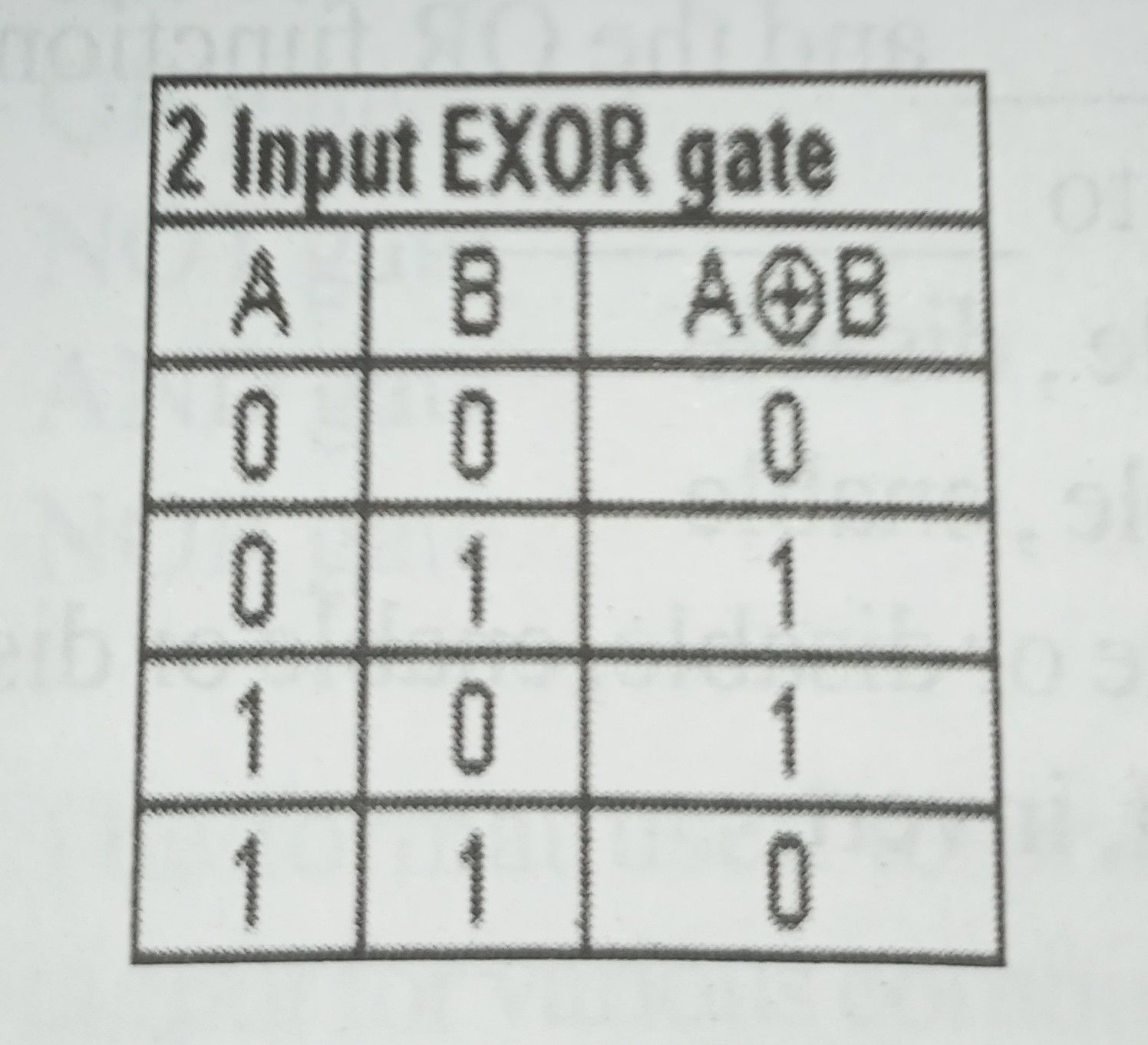NAND GATE

The NAND gate operates as an AND gate followed by a NOT gate. It acts in the manner of the logical operation “and” followed by negation. The output is “false” if both inputs are “true.” Otherwise, the output is “true.”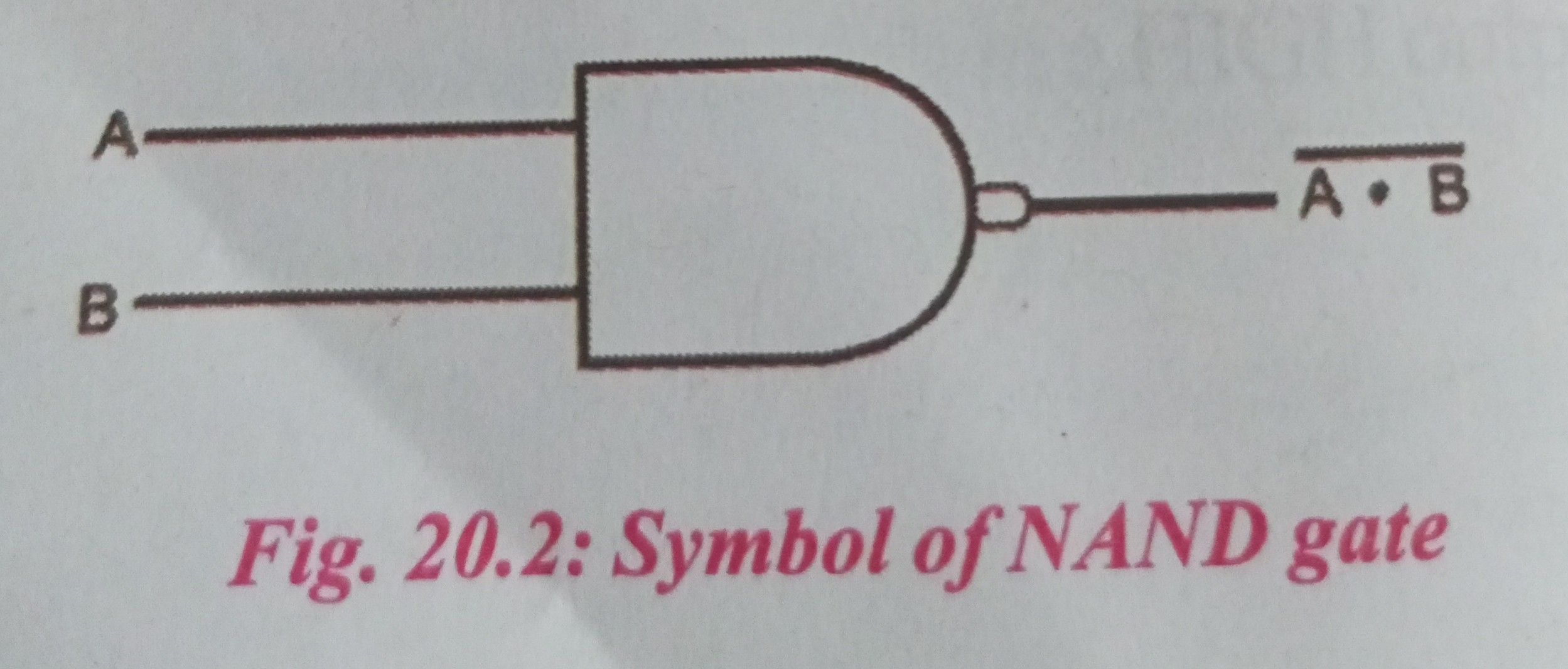This is a NOT-AND gate which is equal to an AND gate followed by a NOT gate. The outputs of all NAND gates are high if any of the inputs are low. The symbol is an AND gate with a small circle on the output. The small circle represents inversion.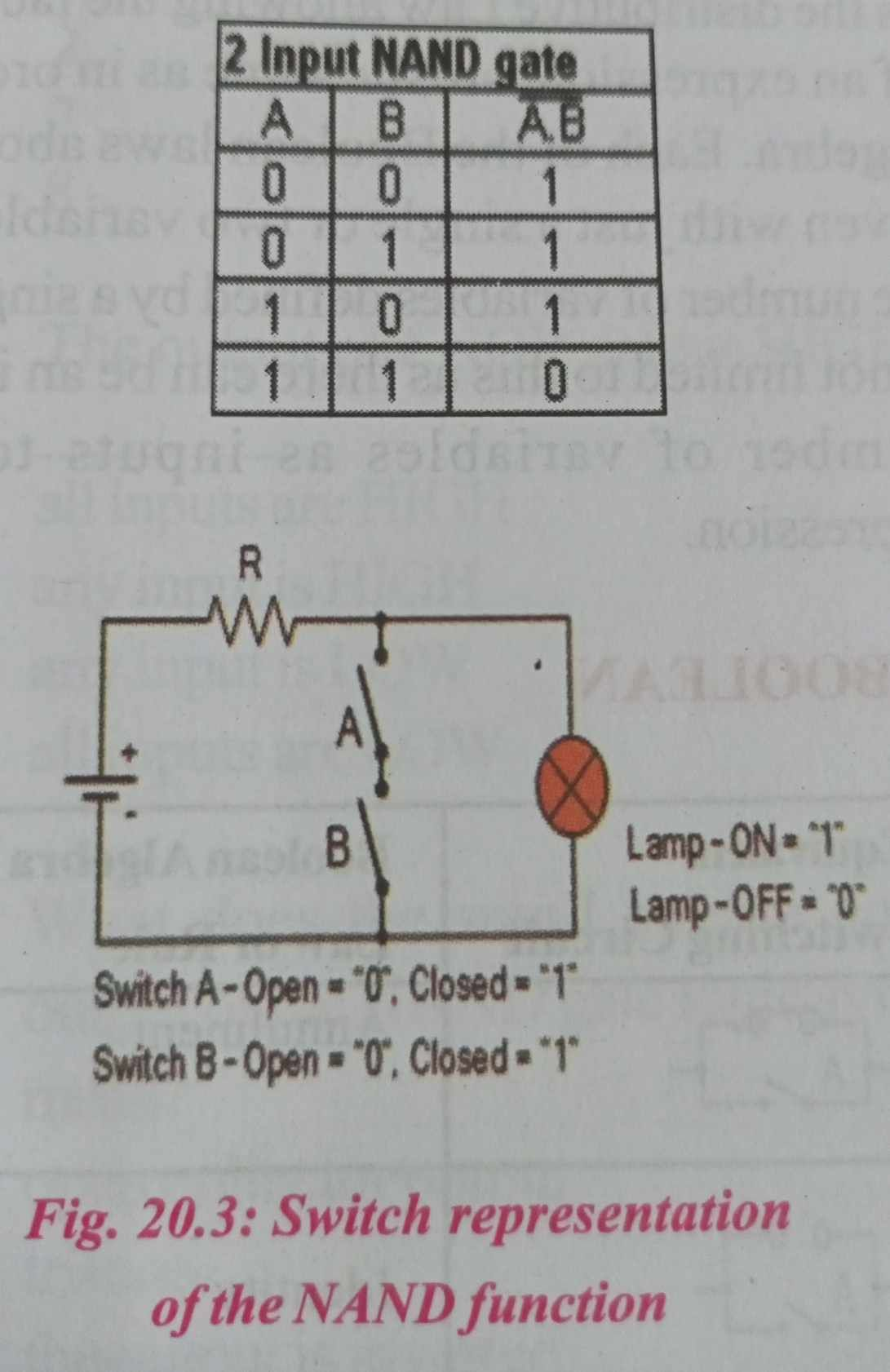NOR GATE

The NOR gate is a combination OR gate followed by an inverter. Its output is “true” if both inputs are “false.” Otherwise, the output is “false.”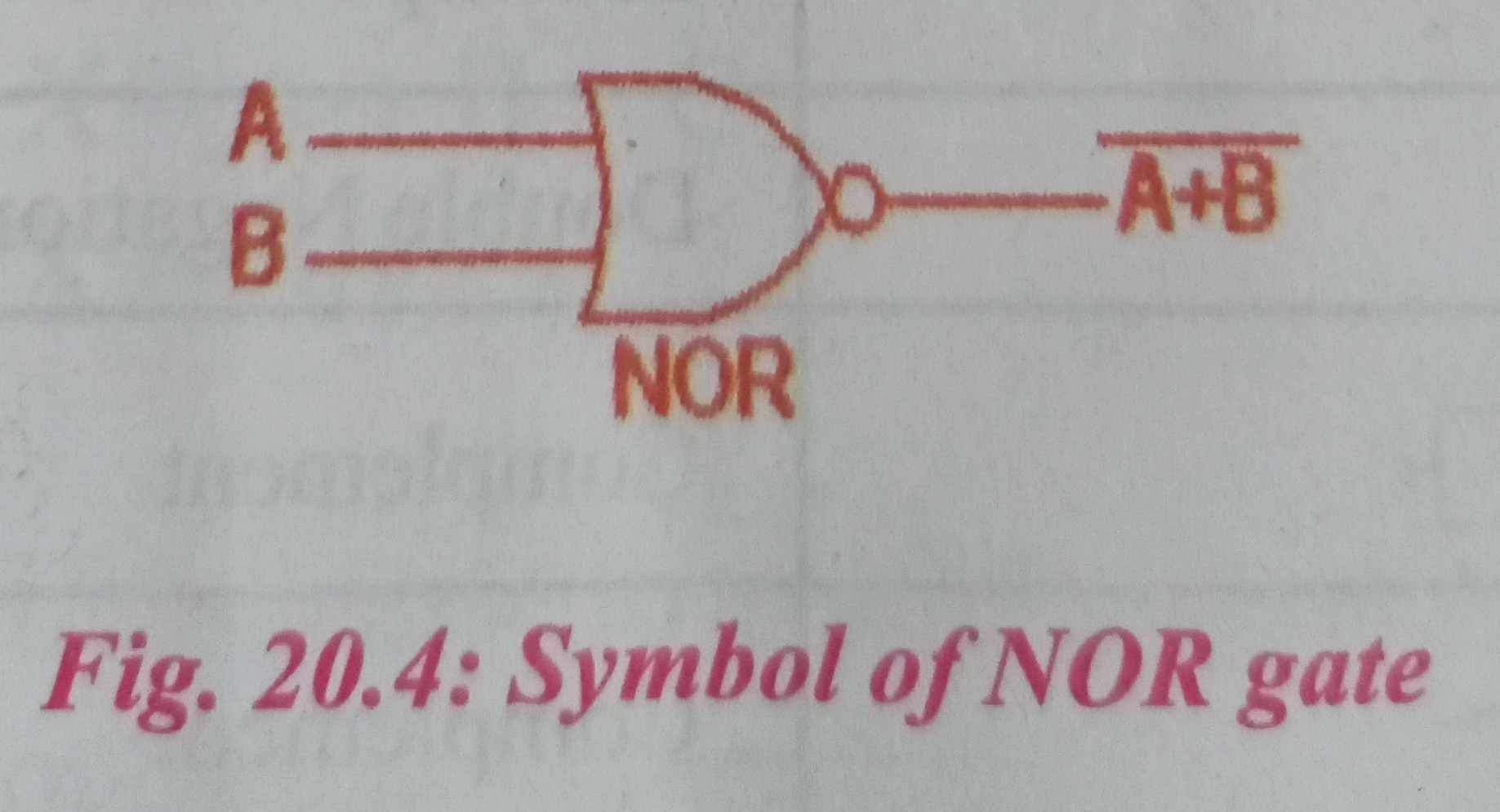This is a NOT-OR gate which is equal to an OR gate followed by a NOT gate. The outputs of all NOR gates are low if any of the inputs are high. The symbol is an OR gate with a small circle on the output. The small circle represents inversion.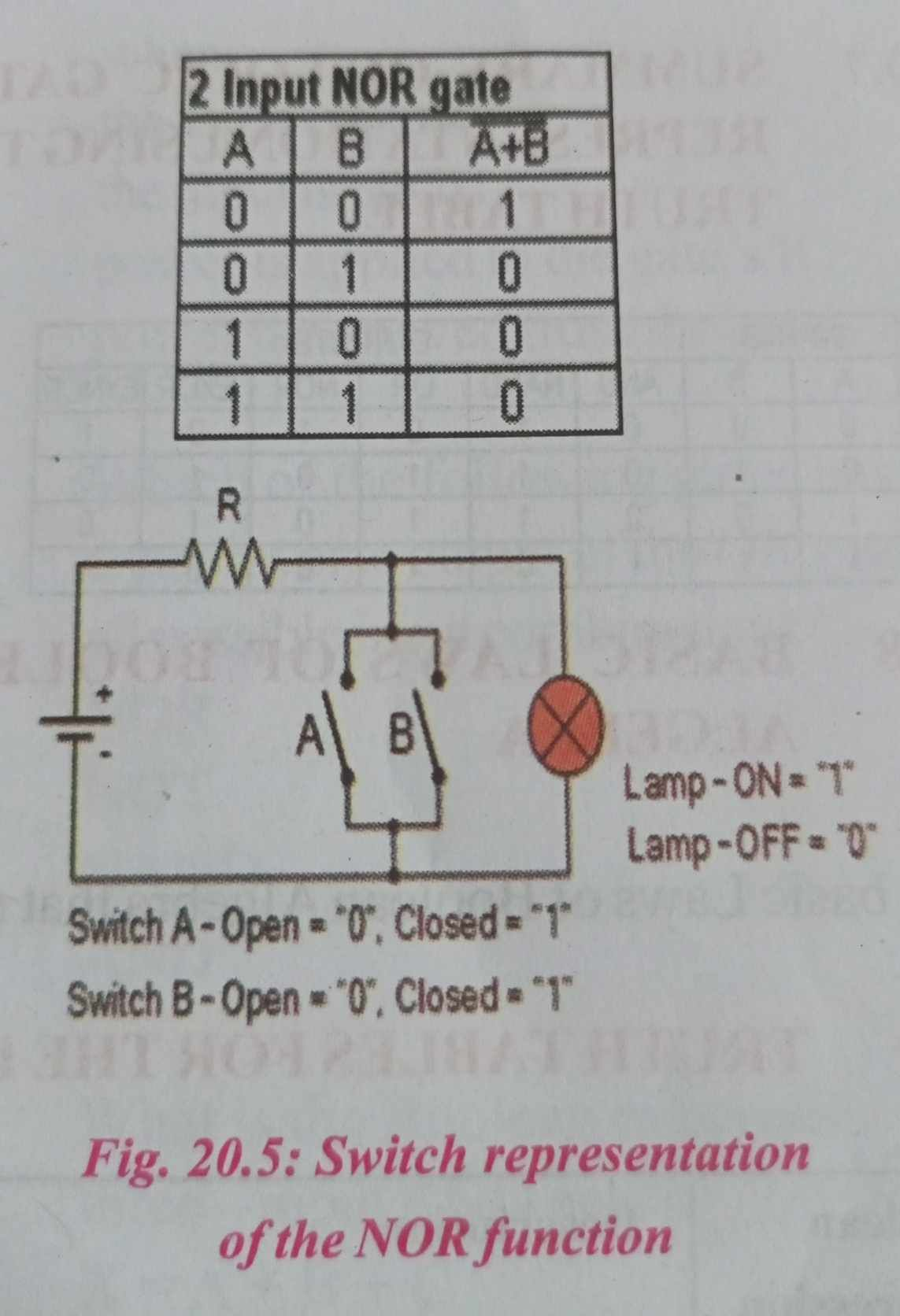EXNOR GATE

The EXNOR (exclusive-NOR) gate is a combination XOR gate followed by an inverter. Its output is “true” if the inputs are the same, and “false” if the inputs are different.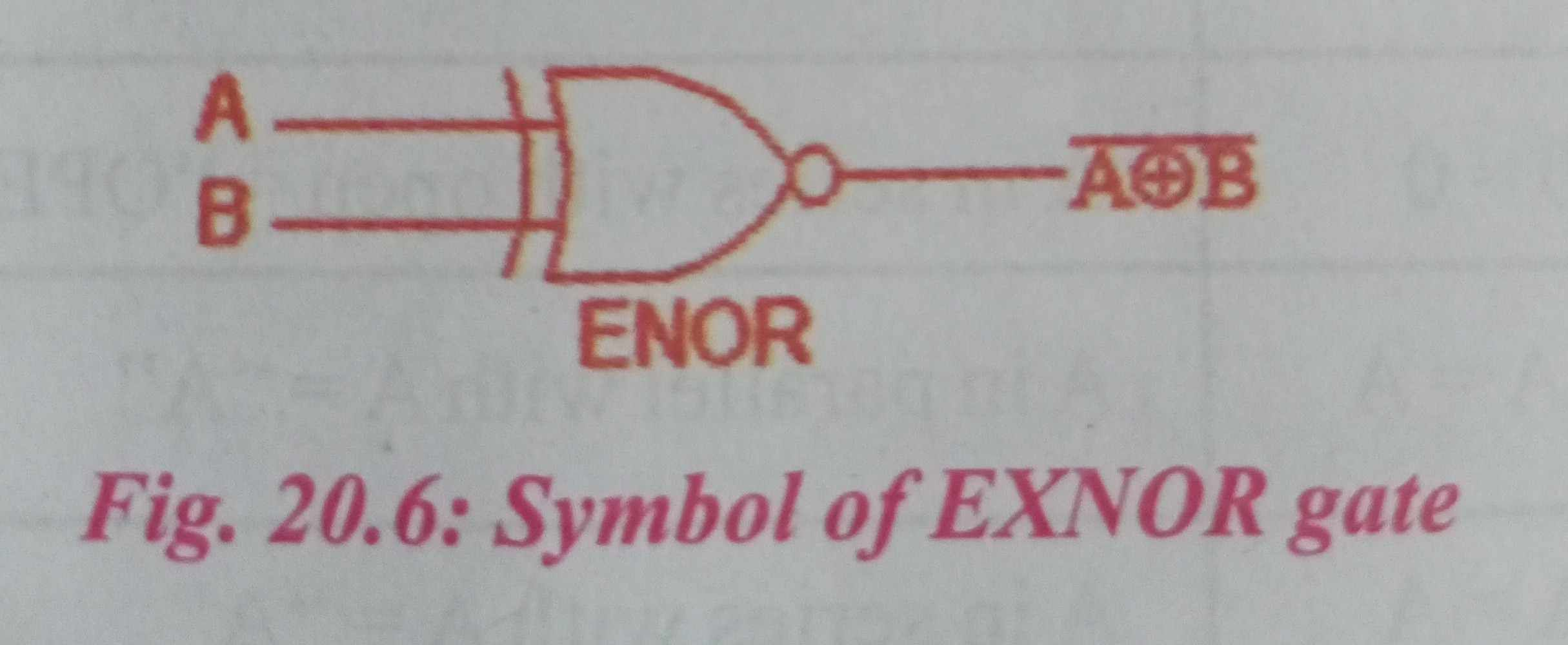The ‘Exclusive-NOR’ gate circuit does the opposite to the EOR gate. It will give a low output if either, but not both, of its two inputs are high. The symbol is an EXOR gate with a small circle on the output. The small circle represents inversion.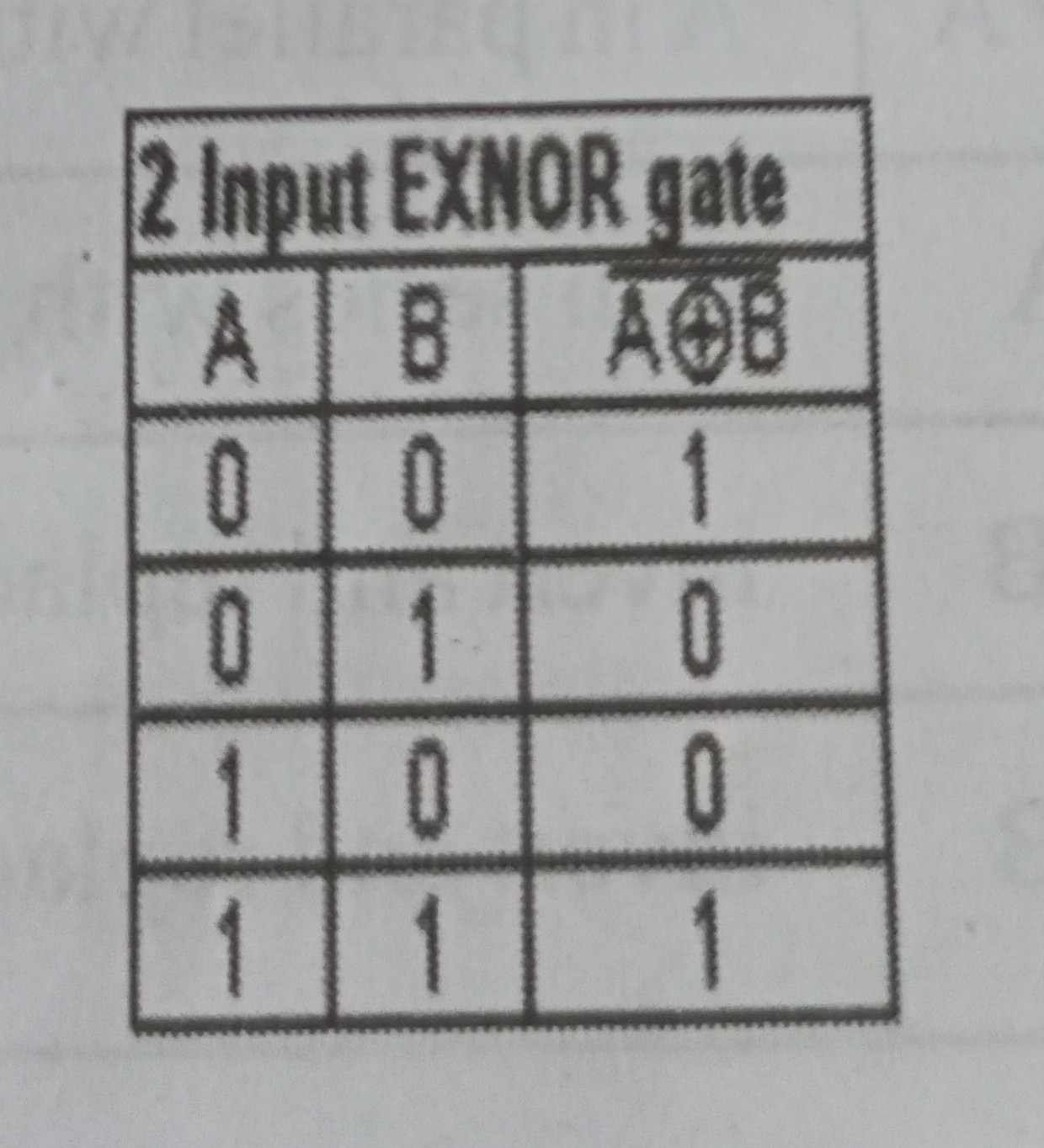SUMMARY OF LOGIC GATES REPRESENTATION USING THE TRUTH TABLE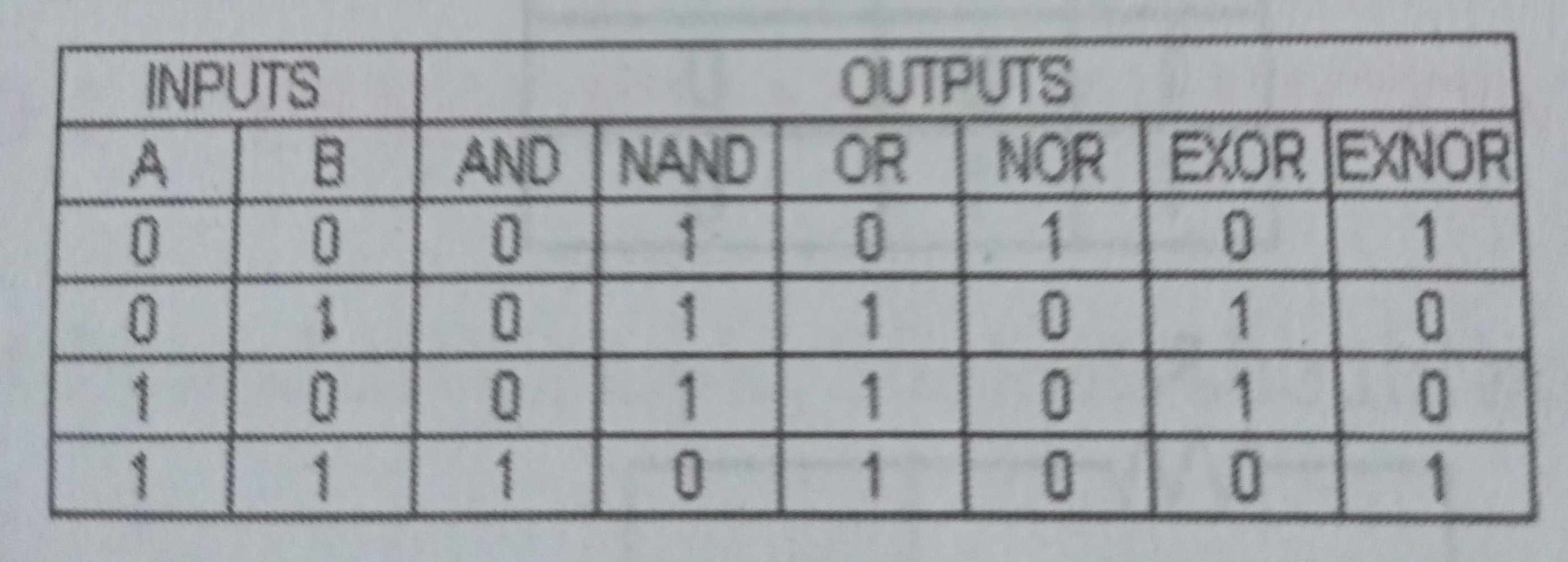BASIC LAWS OF BOOLEAN ALGEBRA

The basic Laws of Boolean Algebra that relate to the Commutative Law allowing a change in position for addition and multiplication, the Associative Law allowing the removal of brackets for addition and multiplication, as well as the distributive Law allowing the factoring of an expression, are the same as in ordinary algebra. Each of the Boolean laws above are given with just a single or two variables, but the number of variables defined by a single law is not limited to this as there can be an infinite number of variables as inputs too the expression.